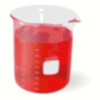#### You may also like### Brimful 2

Which of these infinitely deep vessels will eventually full up?

# Brimful

### Why do this problem?

This problem is an exploration into volumes of revolution. The numbers involved are awkward, which will encourage accurate and careful algebra. By thinking about the volumes and the formula students will gain an insight into the types of functions for which a volume of revolution calculation is possible.

### Possible approach

This question involves 'awkward' numbers, so might be a good problem to use when practising careful integration. Note that the 'usual' volume of revolution formula is for revolution about the $x$ axis, whereas this problem involves revolution about the $y$ axis. Be sure that students are aware of this problem, which might be overcome in a group discussion.

### Key questions

What is the problem with implementing the usual volume of revolution formula?

### Possible extension

Can students explicitly invent a curve which, when revolved, gives a depth of $1$cm when the vessel is half full?

See the related problem Brimful 2

### Possible support

This question is computationally involved. If your students are struggling with the numbers and algebra, focus on the first two curves. You might also change the question so that the curves pass through $(1,1)$ instead of $(2.5,10)$.